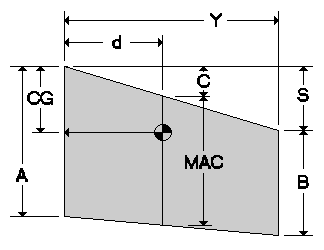Center of Gravity Calculator

 How to use the calculator Input your root chord Input your tip chord Input the sweep distance (see the picture below) Input the wing half span (1/2 the wingspan) Input the desired balance point percentage. (for normal planes it should be between 30-33%, flying wings will be 20-25%) This should get you in the ballpark. Other factors that will effect CG position are tail area, tail moment and airfoils. Always set your plane up a little nose heavy for first flights!!! The calculator will give you the CG at the wing root! Plus a few other numbers you might be interested in.
Simple calculator for determining The CG distance from Leading edge at the root chords when the percent MAC is known.
 Enter Root Chord (A):Enter Tip Chord (B): Enter Sweep Distance (S): Enter Half Span (Y): *Enter %MAC Balance Point: Sweep Distance @ MAC (C) = Mean Aerodynamic Chord (MAC) = MAC Distance from Root (d) = Balance Point @ Root Chord (CG) =

*Note: A 25% of MAC is generally accepted as a good starting point for the CG of a conventional tailed aircraft. The normal range most often falls between 25-30% of MAC

Use this calculator to determine percent of MAC for a given CG

 Enter Root Cord (A):Enter Tip Cord (B): Enter Sweep Distance (S): Enter Half Span (Y): Enter Center of Gravity (CG): Sweep Distance @ MAC (C) = Mean Aerodynamic Cord (MAC) = MAC Distance from Root (d) = % Mean Aerodynamic Cord (%MAC) =
 For S<>0 Forward or Rearward Swept For S=0 Straight Leading Edge C = (S(A+2B)) / (3(A+B)) C = Zero MAC = A-(AC/S)+(BC/S) MAC = A-(2(A-B)(0.5A+B) / (3(A+B))) d = YC / S d = (2Y(0.5A+B)) / (3(A+B)) For all Values of S CG = %MAC B.P.*(MAC) + C

Developed by Geistware of Indiana